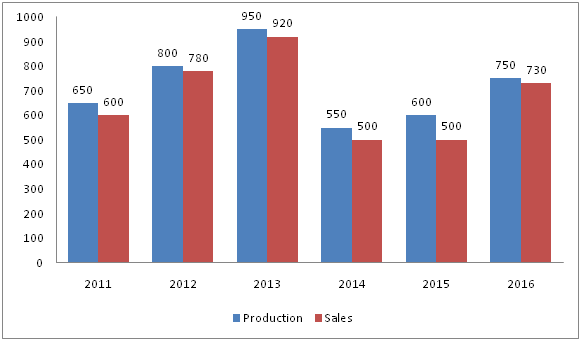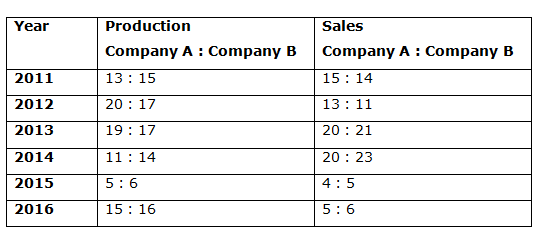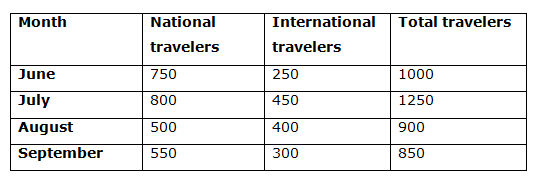# Quantitative Aptitude Questions (Data Interpretation) for RRB PO Manis 2018 Day-186

Dear Readers, IBPS RRB is conducting Online Examination for the recruitment of PO. To enrich your preparation here we have providing new series of Data Interpretation – Quantitative Aptitude Questions. Candidates those who are appearing in RRB PO Mains  Exams can practice these Quantitative Aptitude average questions daily and make your preparation effective.

[WpProQuiz 3522]

Click “Start Quiz” to attend these Questions and view Solutions

Directions (1 – 5): Study the following information carefully and answer the given questions. The data is related to the number of travelers (National + International) handled by the travelers company in 4 months in a certain year.

There are national and international travelers. The total number of national travelers in June is 3/5th of total number of travelers in July. Total number of travelers in July is 1250. The ratio of travelers in June to that of July is 4: 5. The total number of national travelers in June is 100 less than the total number of travelers in September. The number of international travelers in July is 36 % of total number of travelers in July. The number of international travelers in August is half of the national travelers in July. The number of travelers in August is 225 % of total number of international travelers in August. The ratio of total number of national travelers to that of international travelers in September is 11: 6.

1) If in the month of October, the total number of travelers is 60 % more than the total number of travelers in August and the ratio of national travelers to international travelers in October is 7 : 3, then find the difference between the total number of national travelers to that of international travelers in October?

a) 524

b) 550

c) 576

d) 592

e) None of these

2) Out of the total number of national travelers (male and female) in June and August together, the ratio of male national travelers to that of female national travelers is 16: 9. Find the total number of female national travelers in June and August together?

a) 450

b) 480

c) 510

d) 530

e) None of these

3) The total number of international travelers in July is approximately what percentage more/less than the total number of national travelers in August?

a) 20 % more

b) 10 % more

c) 20 % less

d) 10 % less

e) 15 % more

4) If the average number of national travelers in May, June, July, August and September together is 610, then find the ratio between the total number of national travelers in May to that of total number of international travelers in September?

a) 4: 3

b) 3: 2

c) 5: 6

d) 7: 5

e) None of these

5) Total number of international travelers in June is what percentage of total number of international travelers in August?

a) 62.5 %

b) 58 %

c) 71.5 %

d) 75 %

e) None of these

Directions (6 – 10): Study the following information carefully and answer the given questions:

The following bar graph shows the production and sales (In tonnes) of company A in different years.The following table shows the ratio of production of Company A to that of B and the ratio of sales of Company A to that of B.6) The total production of Company A and B together in 2011 and 2013 is approximately what percentage more/less than the total production of company A and B together in 2012 and 2015?

a) 5 % more

b) 15 % less

c) 5 % less

d) 15 % more

e) 20 % less

7) Find the difference between the total sales of Company A in 2012, 2014 and 2016 together to that of total sales of company B in 2011, 2013 and 2015 together?

a) 147 tonnes

b) 141 tonnes

c) 153 tonnes

d) 162 tonnes

e) None of these

8) Find the average sales of company A in all the given years together except the year 2015?

a) 712 tonnes

b) 694 tonnes

c) 706 tonnes

d) 715 tonnes

e) None of these

9) Find the ratio between the total production of company A in 2012, 2014 and 2016 together to that of the total production of company B in the same years?

a) 105 : 109

b) 75 : 82

c) 15 : 17

d) 23 : 26

e) None of these

10) The total sales of company A in 2011 and 2013 together is approximately what percentage of total sales of company B in 2012 and 2014 together?

a) 90 %

b) 105 %

c) 75 %

d) 125 %

e) 140 %

Directions (1 – 5):

Total number of travelers in July = 1250

The total number of national travelers in June = (3/5)*total number of travelers in July

= > (3/5)*1250 = 750

The ratio of travelers in June to that of July = 4: 5 (4x, 5x)

5x = 1250

X = 250

Total number of travelers in June = 4x = 1000

The total number of national travelers in June = 100 less than the total number of travelers in September

= > 750 = Total travelers in September – 100

Total number of travelers in September = 750 + 100 = 850

The number of international travelers in July = (36/100)*total number of travelers in July.

The number of international travelers in July = (36/100)*1250 = 450

The number of national travelers in July = 1250 – 450 = 800

The number of international travelers in August = (1/2)*National travelers in July

= > (1/2)*800 = 400

The number of travelers in August = (225/100)*total number of international travelers in August

= > (225/100)*400 = 900

The number of national travelers in August = 900 – 400 = 500

The ratio of total number of national travelers to that of international travelers in September = 11 : 6 (11x, 6x)

17x = 850

X = 50

The total number of national travelers in September = 11x = 550

The total number of international travelers in September = 6x = 300Direction (1-5) :

The total number of travelers in October = (160/100)*the total number of travelers in August

= > (160/100)*900 = 1440

The ratio of national travelers to international travelers in October

= > 7 : 3 (7x, 3x)

10x = 1440

X = 144

The difference between the total number of national travelers to that of international travelers in October

= > 4x = 144*4 = 576

The total number of national travelers (male and female) in June and August together

= > 750 + 500 = 1250

The ratio of male national travelers to that of female national travelers

= > 16 : 9 (16x, 9x)

25x = 1250

X = 50

The total number of female national travelers in June and August together

= > 9x = 9*50 = 450

The total number of international travelers in July = 450

The total number of national travelers in August = 500

Required % = [(500 – 450)/500]*100 = 10 % less

The average number of national travelers in May, June, July, August and September together = 610

The total number of national travelers in May, June, July, August and September together = 610*5 = 3050

The total number of national travelers in May

= > 3050 – (750 + 800 + 500 + 550) = 450

The total number of international travelers in September = 300

Required ratio = 450: 300 = 3: 2

Total number of international travelers in June = 250

Total number of international travelers in August = 400

Required % = (250/400)*100 = 62.5 %

Direction (6-10) :

The total production of Company A and B together in 2011 and 2013

= > 650 + 650*(15/13) + 950 + 950*(17/19)

= > 650 + 750 + 950 + 850 = 3200 tonnes

The total production of company A and B together in 2012 and 2015

= > 800 + 800*(17/20) + 600 + 600*(6/5)

= > 800 + 680 + 600 + 720 = 2800 tonnes

Required % = [(3200 – 2800)/2800]*100 = 14.28 % = 15 % more

The total sales of Company A in 2012, 2014 and 2016 together

= > 780 + 500 + 730 = 2010 tonnes

The total sales of company B in 2011, 2013 and 2015 together

= > 600*(14/15) + 920*(21/20) + 500*(5/4)

= > 560 + 966 + 625 = 2151 tonnes

Required difference = 2151 – 2010 = 141 tonnes

The average sales of company A in all the given years together

= > [600 + 780 + 920 + 500 + 730]/65

= > 3530/5 = 706 tonnes

The total production of company A in 2012, 2014 and 2016 together

= > 800 + 550 + 750 = 2100 tonnes

The total production of company B in 2012, 2014 and 2016 together

= > 800*(17/20) + 550*(14/11) + 750*(16/15)

= > 680 + 700 + 800 = 2180 tonnes

Required ratio = 2100 : 2180 = 105 : 109

The total sales of company A in 2011 and 2013 together

= > 600 + 920 = 1520 tonnes

The total sales of company B in 2012 and 2014 together

= > 780*(11/13) + 500*(23/20)

= > 660 + 575 = 1235 tonnes

Required % = (1520/1235)*100 = 123 % = 125 %

Daily Practice Test Schedule | Good Luck

 Topic Daily Publishing Time Daily News Papers & Editorials 8.00 AM Current Affairs Quiz 9.00 AM Quantitative Aptitude “20-20” 11.00 AM Vocabulary (Based on The Hindu) 12.00 PM General Awareness “20-20” 1.00 PM English Language “20-20” 2.00 PM Reasoning Puzzles & Seating 4.00 PM Daily Current Affairs Updates 5.00 PM Data Interpretation / Application Sums (Topic Wise) 6.00 PM Reasoning Ability “20-20” 7.00 PM English Language (New Pattern Questions) 8.00 PM